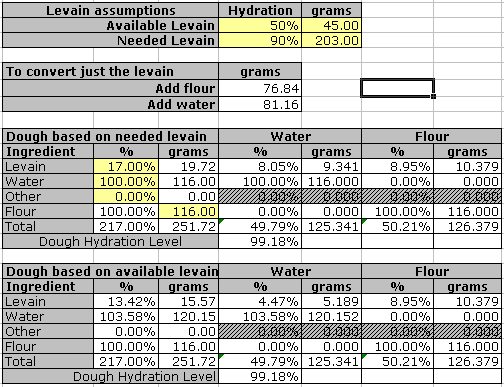• Home
• Calculator Sheets
• Dough Topics

# Introduction

Levain (french for "leaven") is a generic term for a preferment. Most of the time when the French use the term they mean what we call a starter, but it could also apply to a poolish, biga or sponge.

All levains contain an amount of flour and water, and sometimes there are additional ingredients. The main difference between various levains is often its water content. A specific formula may call for the use of a levain with a specified water content, but you may not have that available. This worksheet helps you calculate how to deal with the situation.

## Levain and hydration

Levains are typically characterized by the type of flour used and their hydration level. The hydration level is defined as the weight of water used divided by the weight of flour used. In terms of baker's percentages, therefore, the hydration level is equal to the baker's percentage of the water in the formula (this is only true for "straight" formulas). When we speak of water content, we mean the percentage of the overall weight that is water. This is not the same as the hydration. An example is a typical "stiff" levain with 50% hydration. It is maintained by adding flour and water to a piece of mature starter in the ratio of 2:1 (2 parts by weight flour to 1 part by weight water). The water content, however, is 50/(100+50) = 33 1/3%.

### Adjusting a levain for different hydration

If you have a levain of a specific hydration level and you want to convert it to another hydration level you start by computing the amount of water and flour in the piece you are going to "feed." You know the hydration level desired for the new levain so if you choose the final weight, you can calculate how much water and flour is needed, in total, for that levain. Subtract what you already have and you will know what you need to add.

An example. Convert a "stiff" levain at 50% hydration to a liquid levain at 90% hydration. Assume we start with 45g and want to make the new levain be 200g. The starting levain contains 150% total, and thus 15 grams of water (45/3) and 30 grams flour. The "new" levain needs to contain 190%, or 90*200/190 = 94.74 grams of water and 100*200/190 = 105.26 grams of flour. That means we need to add 105.26-30 = 75.26 grams of flour and 94.74-15 = 79.74 grams of water.

If you don't like the idea of making big adjustments to a levain, just do it in steps. In the above example, at one feeding you could go from 50% hydration to 70%, and in the next you could go from 75% to 90%.

### Adjusting a levain in a two stage build

Often times the formula for a bread calls for the use of a particular starting levain to build a specific levain for this bread as a preferment, which then goes into the final dough. If your available starter does not hav the correct hydration you compensate by adding or removing water from the rest of the build levain appropriately. The principle is the same as for adjusting a levain by itself, except that this time to target weight for the built levain is determined by the formula, and their may be other ingredients.

### How to use the worksheet

You will find the following on the "Levains" worksheet (this assumes that levains only contain flour and water):As usual, the yellow cells is where you enter information. Shown here is an example of an availabe levain at 50% hydration, weighing 45 grams, and a needed levain at 100%, weighing 203 grams.

If you just wish to convert the levain, and feed at the seem time, to arrive at the desired weight and hydration you will need to add 76.84g of flour and 81.16g of water.

The second part illustrates a more common scenario. You maintain a 50% hydration starter and now you are preparing to make a bread that requires building a levain (possible with different kind of flour) that is of a different hydration. The first block is where you input the percentages for the overall desired levain (based on 17% existing levain and 100% hydration in this step). The second blocks shows how to adjust the formula to make it work with the levain you have. The amount of flour contributed from the levain is equal in both cases, so the adjustments are made in the dough by adjusting additional flour and additional water.